## Osculating Circle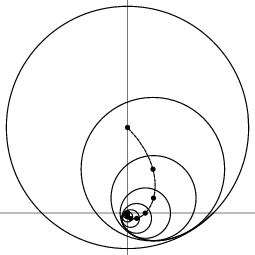The Circle which shares the same Tangent as a curve at a given point. The Radius of Curvature of the osculating circle is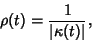(1)

where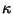is the Curvature, and the center is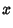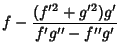(2)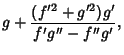(3)

i.e., the centers of the osculating circles to a curve form the Evolute to that curve.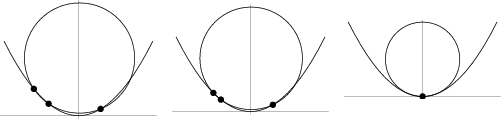In addition, let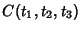denote the Circle passing through three points on a curve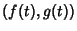with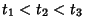. Then the osculating circle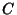is given by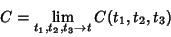(4)

(Gray 1993).

Gardner, M. The Game of Life, Parts I-III.'' Chs. 20-22 in Wheels, Life, and other Mathematical Amusements. New York: W. H. Freeman, pp. 221, 237, and 243, 1983.
Gray, A. Osculating Circles to Plane Curves.'' §5.6 in Modern Differential Geometry of Curves and Surfaces. Boca Raton, FL: CRC Press, pp. 90-95, 1993.